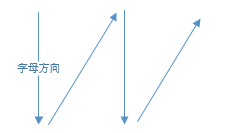# [LeetCode] 6、Z 字形变换

## 题目描述

L   C   I   R
E T O E S I I G
E   D   H   N


## 解题思路• 模拟遍历法：（算法实现的关键是“方向转换”，见代码）
• 字符串 s 是以 $Z$ 字形为顺序存储的字符串，目标是按行打印。
• numRows 行字符串分别为 $s_1 , s_2 ,..., s_n$，则容易发现：按顺序遍历字符串 s 时，每个字符 c$Z$ 字形中对应的 行索引 先从 $s_1$ 增大至 $s_n$，再从 $s_n$ 减小至 $s_1$ …… 如此反复。
• 因此，解决方案为：模拟这个行索引的变化，在遍历 s 时把每个字符填到正确的行 res[i]

## 参考代码

class Solution {
public:
string convert(string str, int numRows) {
if(numRows == 1)
return str;

vector<string> tempRes(numRows, "");
int i = 0, flag = -1;
for(auto c: str){
tempRes[i] += c;
if(i == 0 || i == numRows - 1)  // 方向转换
flag = -flag;
i += flag;
}

string res = "";
for(auto s: tempRes)
res += s;
return res;
}
};


©️2019 CSDN 皮肤主题: 大白 设计师: CSDN官方博客